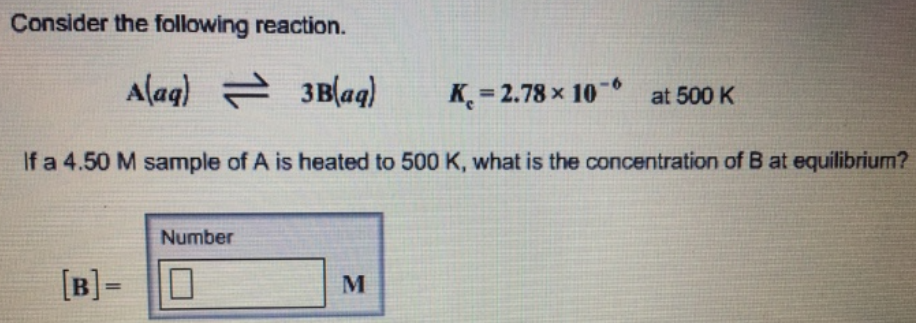# Problem: Consider the following reaction. A (aq) ⇌ 3B (aq)      Kc = 2.78 x 10-6 at 500 K If a 4.50 M sample of A is heated to 500 K, what is the concentration of B at equilibrium?

###### FREE Expert Solution###### Problem Details

Consider the following reaction.

A (aq) ⇌ 3B (aq)      Kc = 2.78 x 10-6 at 500 K

If a 4.50 M sample of A is heated to 500 K, what is the concentration of B at equilibrium?